# Pole and polar

"Polar line" redirects here. For the railway line, see Polar Line.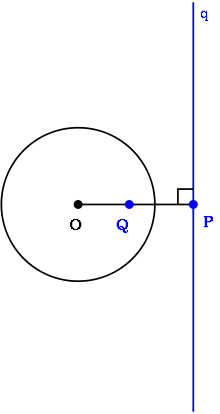The polar line q to a point Q with respect to a circle of radius r centered on the point O. The point P is the inversion point of Q; the polar is the line through P that is perpendicular to the line containing O, P and Q.

In geometry, the pole and polar are respectively a point and a line that have a unique reciprocal relationship with respect to a given conic section. If the point lies on the conic section, its polar is the tangent line to the conic section at that point.

For a given circle, reciprocation in a circle means the transformation of each point in the plane into its polar line and each line in the plane into its pole.

## Properties

Poles and polars have several useful properties:

• If a point P lies on a line l, then the pole L of the line l lies on the polar p of point P.
• If a point P moves along a line l, its polar p rotates about the pole L of the line l.
• If two tangent lines can be drawn from a pole to the conic section, then its polar passes through both tangent points.
• If a point lies on the conic section, its polar is the tangent through this point to the conic section.
• If a point P lies on its own polar line, then P is on the conic section.
• Each line has, with respect to a non-degenerated conic section, exactly one pole.

## Special case of circles

The pole of a line L in a circle C is a point P that is the inversion in C of the point Q on L that is closest to the center of the circle. Conversely, the polar line (or polar) of a point P in a circle C is the line L such that its closest point Q to the center of the circle is the inversion of P in C.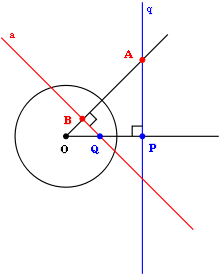If a point A lies on the polar line q of another point Q, then Q lies on the polar line a of A. More generally, the polars of all the points on the line q must pass through its pole Q.

The relationship between poles and polars is reciprocal. Thus, if a point Q is on the polar line A of a point P, then the point P must lie on the polar line B of the point Q. The two polar lines A and B need not be parallel.

There is another description of the polar line of a point P in the case that it lies outside the circle C. In this case, there are two lines through P which are tangent to the circle, and the polar of P is the line joining the two points of tangency (not shown here). This shows that pole and polar line are concepts in the projective geometry of the plane and generalize with any nonsingular conic in the place of the circle C.

## Reciprocation and projective duality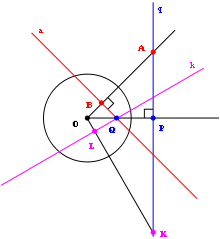Illustration of the duality between points and lines, and the double meaning of "incidence". If two lines a and k pass through a single point Q, then the polar q of Q joins the poles A and K of the lines a and k, respectively.

The concepts of a pole and its polar line were advanced in projective geometry. For instance, the polar line can be viewed as the set of projective harmonic conjugates of a given point, the pole, with respect to a conic. The operation of replacing every point by its polar and vice versa is known as a polarity.

A polarity is a correlation that is also an involution.

## General conic sections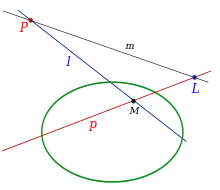Line p is the polar line to point P, l to L and m to M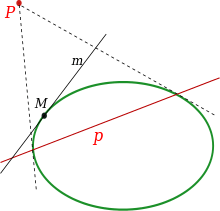p is the polar line to point P ; m is the polar line to M

The concepts of pole, polar and reciprocation can be generalized from circles to other conic sections which are the ellipse, hyperbola and parabola. This generalization is possible because conic sections result from a reciprocation of a circle in another circle, and the properties involved, such as incidence and the cross-ratio, are preserved under all projective transformations.

### Calculating the polar of a point

A general conic section may be written as a second-degree equation in the Cartesian coordinates (x, y) of the planewhere Axx, Axy, Ayy, Bx, By, and C are the constants defining the equation. For such a conic section, the polar line to a given pole point (ξ, η) is defined by the equationwhere D, E and F are likewise constants that depend on the pole coordinates (ξ, η)### Calculating the pole of a line

The pole of the line, relative to the non-degenerated conic sectioncan be calculated in two steps.

First, calculate the numbers x, y and z fromNow, the pole is the point with coordinates## Properties

Poles and polars have several useful properties.

If a point P lies on a line l, then the pole L of the line l lies on the polar p of point P.

If a point P moves along a line l, its polar p rotates about the pole L of the line l.

If two tangent lines can be drawn from a pole to the conic section, then its polar passes through both tangent points.

If a point lies on the conic section, its polar is the tangent through this point to the conic section.

If a point P lies on its own polar line, then P is on the conic section.

Each line has, with respect to a non-degenerated conic section, exactly one pole.

## Applications

Poles and polars were defined by Joseph Diaz Gergonne and play an important role in his solution of the problem of Apollonius.

In planar dynamics a pole is a center of rotation, the polar is the force line of action and the conic is the mass–inertia matrix. The pole–polar relationship is used to define the center of percussion of a planar rigid body. If the pole is the hinge point, then the polar is the percussion line of action as described in planar screw theory.Wikimedia Commons has media related to Poles and polars.